# 模型的建立与评估

``````import pandas as pd
import numpy as np
import seaborn as sns
import matplotlib.pyplot as plt
``````
``````%matplotlib inline
``````
``````plt.rcParams['font.sans-serif'] = ['SimHei']  # 用来正常显示中文标签
plt.rcParams['axes.unicode_minus'] = False  # 用来正常显示负号
plt.rcParams['figure.figsize'] = (10, 6)  # 设置输出图片大小
``````
``````# 读取训练数集
train.shape
``````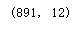``````train.head()
``````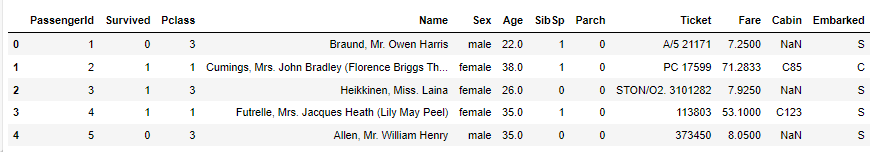# 1. 特征工程

## 1.1 缺失值填充

• 对分类变量缺失值：填充某个缺失值字符(NA)、用最多类别的进行填充
• 对连续变量缺失值：填充均值、中位数、众数
``````# 对分类变量进行填充
train['Cabin'] = train['Cabin'].fillna('NA')
train['Embarked'] = train['Embarked'].fillna('S')
# 对连续变量进行填充
train['Age'] = train['Age'].fillna(train['Age'].mean())
# 检查缺失值比例
train.isnull().sum().sort_values(ascending=False)
``````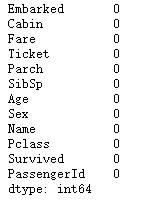## 1.2 编码分类变量

``````# 取出所有的输入特征
data = train[['Pclass','Sex','Age','SibSp','Parch','Fare', 'Embarked']]
# 进行虚拟变量转换
data = pd.get_dummies(data)
``````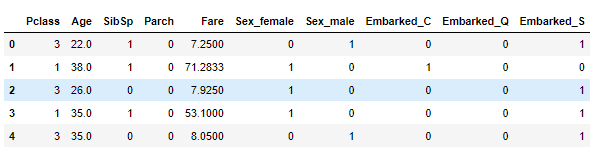# 2. 模型搭建

• 处理完前面的数据我们就得到建模数据，下一步是选择合适模型。
• 在进行模型选择之前我们需要先知道数据集最终是进行监督学习还是无监督学习，监督学习需要数据有标签。
• 除了根据我们任务来选择模型外，还可以根据数据样本量以及特征的稀疏性来决定，样本量多可以选择复杂的模型，如深度学习。
• 刚开始我们总是先尝试使用一个基本的模型来作为其baseline，进而再训练其他模型做对比，最终选择泛化能力或性能比较好的模型。
• 建模前需要判断数据是否为同一分布，机器学习的基本假设是数据的训练集、验证集和预测集基本属于同一分布。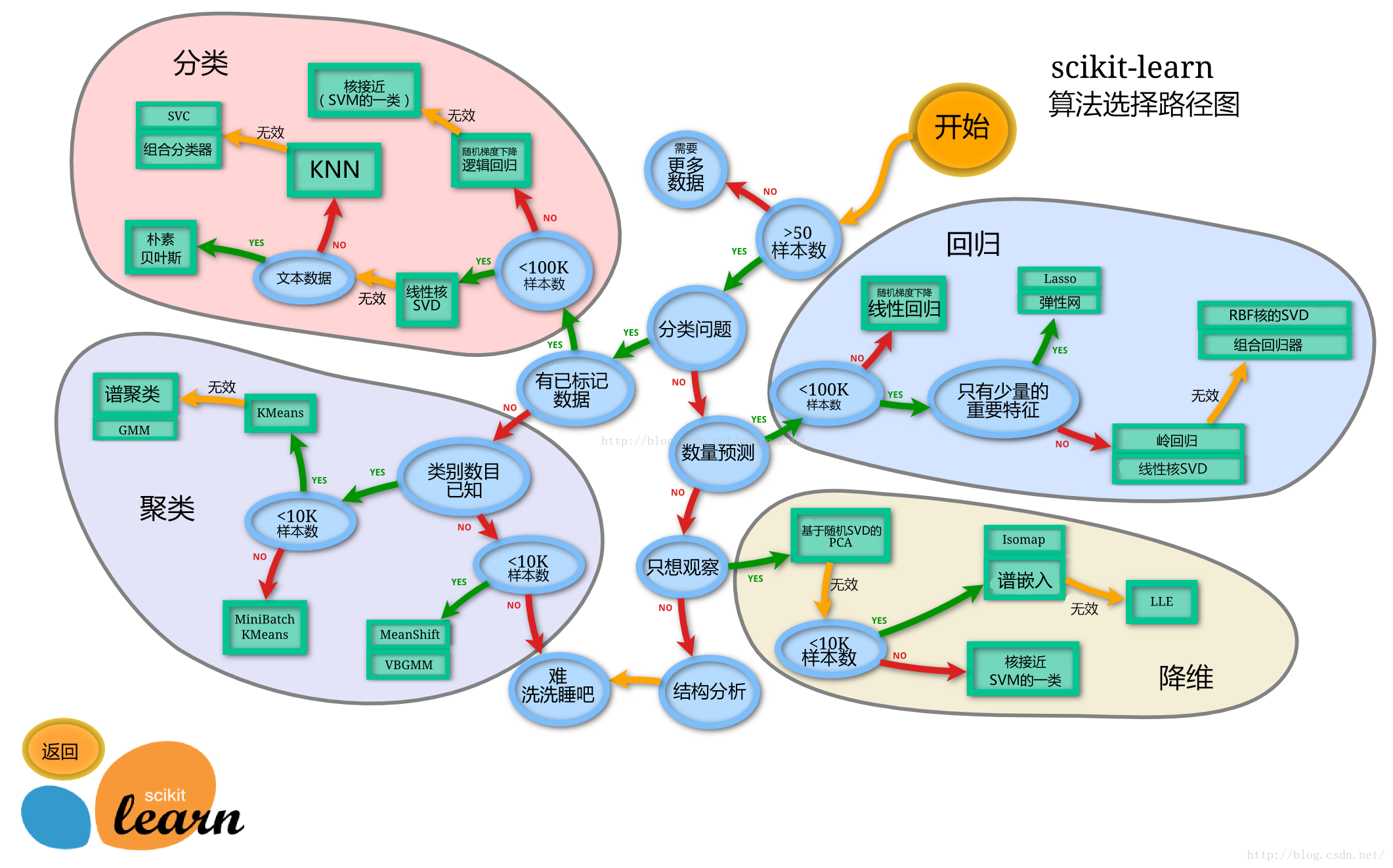## 2.1 切割训练集和测试集

• 按比例切割训练集和测试集
• 按目标变量分层进行等比切割
• 设置随机种子以便结果能复现
• 切割数据集是为了后续能评估模型泛化能力
• sklearn中切割数据集的方法为train_test_split
• 查看函数文档可以在jupyter noteboo里面使用train_test_split?后回车即可看到

## 什么情况下切割数据集的时候不用进行随机选取？

• 时序数据集一般不进行随机选取，而是分段切割，避免用未来的数据预测历史数据，发生数据泄露
• 当数据类别不均衡时，也不进行随机选取，会采用重采样，以减轻类别不均衡现象。
``````from sklearn.model_selection import train_test_split
# 一般先取出X和y后再切割，有些情况会使用到未切割的，这时候X和y就可以用
X = data
y = train['Survived']
# 对数据集进行切割
X_train, X_test, y_train, y_test = train_test_split(X, y, stratify=y, random_state=0)
# 查看数据形状
X_train.shape, X_test.shape
``````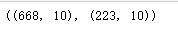## 2.2 模型创建

• 创建基于线性模型的分类模型（逻辑回归）
• 创建基于树的分类模型（决策树、随机森林）
• 查看模型的参数，并更改参数值，观察模型变化

## 对于多分类问题，线性模型是怎么进行分类的？

``````from sklearn.linear_model import LogisticRegression
from sklearn.ensemble import RandomForestClassifier
# 默认参数逻辑回归模型
lr = LogisticRegression()
lr.fit(X_train, y_train)
# 查看训练集和测试集score值
print("Training set score: {:.2f}".format(lr.score(X_train, y_train)))
print("Testing set score: {:.2f}".format(lr.score(X_test, y_test)))
``````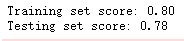``````# 默认参数的随机森林分类模型
rfc = RandomForestClassifier()
rfc.fit(X_train, y_train)
print("Training set score: {:.2f}".format(rfc.score(X_train, y_train)))
print("Testing set score: {:.2f}".format(rfc.score(X_test, y_test)))
``````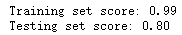## 2.3 输出模型预测结果

• 输出模型预测分类标签
• 输出不同分类标签的预测概率
• 一般监督模型在sklearn里面有个predict能输出预测标签，predict_proba则可以输出标签概率

## 预测标签的概率对我们有什么帮助？

``````# 预测标签
pred = lr.predict(X_train)
# 此时我们可以看到0和1的数组
pred[:10]
``````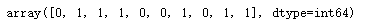``````# 预测标签概率
pred_proba = lr.predict_proba(X_train)
pred_proba[:10]
``````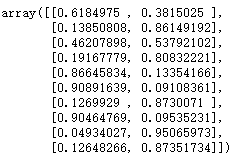# 3. 模型评估

• 模型评估是为了知道模型的泛化能力。
• 交叉验证（cross-validation）是一种评估泛化性能的统计学方法，它比单次划分训练集和测试集的方法更加稳定、全面。
• 在交叉验证中，数据被多次划分，并且需要训练多个模型。
最常用的交叉验证是 k 折交叉验证（k-fold cross-validation），其中 k 是由用户指定的数字，通常取 5 或 10。
• 准确率（precision）度量的是被预测为正例的样本中有多少是真正的正例
• 召回率（recall）度量的是正类样本中有多少被预测为正类
• f-分数是准确率与召回率的调和平均

## 3.1 交叉验证

• 用10折交叉验证来评估逻辑回归模型
• 计算交叉验证精度的平均值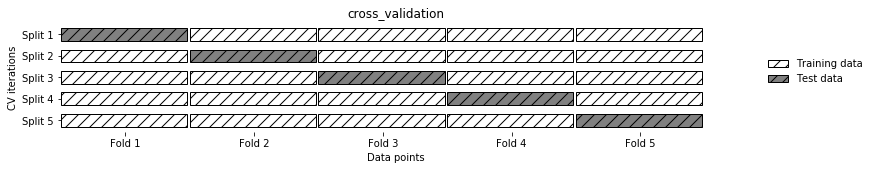## k折越多的情况下会带来什么样的影响？

K折越多，单次训练验证时，用作训练集的数据就会越多，而用作验证集的数据越少。这样平均的结果会更加可靠，但是所耗费的总时间也会增多。

``````from sklearn.model_selection import cross_val_score
lr = LogisticRegression(C=100)
scores = cross_val_score(lr, X_train, y_train, cv=10)
scores
``````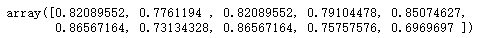``````# 平均交叉验证分数
print("Average cross-validation score: {:.2f}".format(scores.mean()))
``````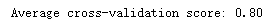## 3.2 混淆矩阵

• 计算二分类问题的混淆矩阵
• 计算精确率、召回率以及f-分数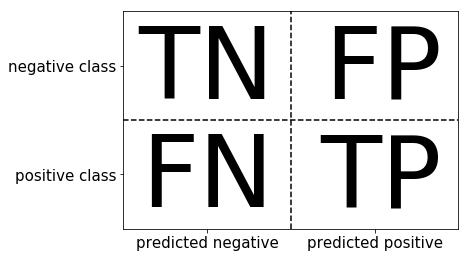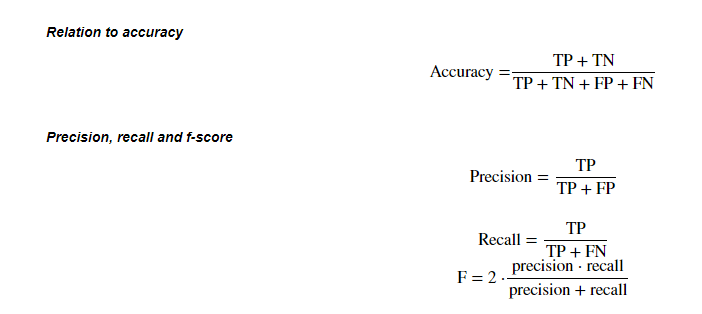``````from sklearn.metrics import confusion_matrix
lr = LogisticRegression(C=100)
lr.fit(X_train, y_train)
pred = lr.predict(X_train)
confusion_matrix(y_train, pred)
``````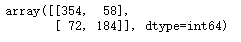``````from sklearn.metrics import classification_report
print(classification_report(y_train, pred))
``````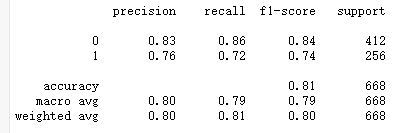## 3.3 ROC曲线

• ROC曲线在sklearn中的模块为sklearn.metrics
• ROC曲线下面所包围的面积越大越好
``````from sklearn.metrics import roc_curve
fpr, tpr, thresholds = roc_curve(y_test, lr.decision_function(X_test))
plt.plot(fpr, tpr, label="ROC Curve")
plt.xlabel("FPR")
plt.ylabel("TPR (recall)")
# 找到最接近于0的阈值
close_zero = np.argmin(np.abs(thresholds))
plt.plot(fpr[close_zero], tpr[close_zero], 'o', markersize=10, label="threshold zero", fillstyle="none", c='k', mew=2)
plt.legend(loc=4)
``````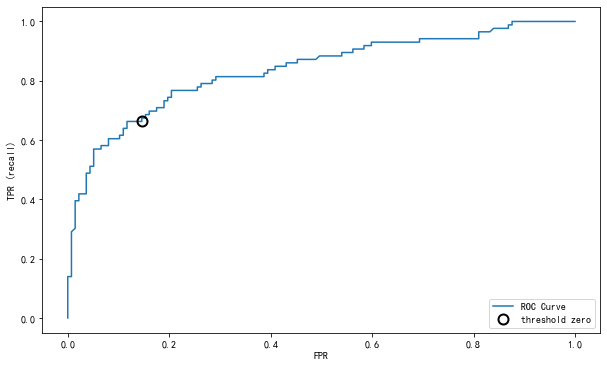## 参考资料

Datawhale 开源文档：https://github.com/datawhalechina/hands-on-data-analysis
https://gitee.com/datawhalechina/hands-on-data-analysis/tree/master